# Drawing with JavaScript in HTML5 vs ActionScript 3 in Flash

HTML5 introduces an element called a "canvas" on which we can use JavaScript to draw. This offers a quick and easy approach to drawing dynamic content. This article will compare this relatively new (and not yet cross-browser compatible) option for drawing to Adobe Flash's ActionScript 3. JavaScript and ActionScript have common roots as they are both dialects of ECMAScript.

For this article we are going to draw something with a little complexity to better compare the two languages. I have chosen a warning icon I made in Photoshop.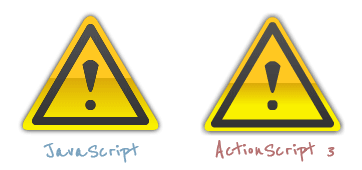## An Initial Difference

As we dive right into the code try not to be too concerned about the math. The point of this article is not to explain the trigonometry behind a triangle, just to compare how we draw the triangle. That said, the first thing we do is declare a few variables.

```var phi = Math.tan((this.width/2) / this.height);
var x = this.innerBorder / Math.cos(phi);
var y = x / Math.tan(phi);
var gamma = Math.sqrt(Math.abs(this.innerBorder*this.innerBorder-x*x));```
```var phi:Number = Math.tan((iconWidth/2) / iconHeight);
var x:Number = innerBorder / Math.cos(phi);
var y:Number = x / Math.tan(phi);
var gamma:Number = Math.sqrt(Math.abs(innerBorder*innerBorder - x*x));```

The code is similar in both languages, but two differences appear immediately and will be consistent throughout this article:

Type Declaration:
JavaScript is loosely typed, so we can't declare a variable's data type, like we can in ActionScript. For example, in the code above: `var phi:Number` declares that variable `phi` is a number. Trying to assign it as anything else will throw an error. It's worth noting that ActionScript 3 does not require type declaration.
Use of the "this" keyword:
In JavaScript you will see properties like `width` preceded by the keyword "this" as in `this.keyword`. You can do this is ActionScript, but it is optional.

## Basic Shape: A Triangular Path

The icon we are drawing has three major components:

Background:
A triangle with rounded corners, a gradient fill, and a subtle shadow.
Inner Border:
A smaller triangle border inside of the background.
Exclamation Point:
A bang character "!" inside the center of the icon.

Since both JavaScript and ActionScript support paths, we will use paths to define our shapes. Let us start with the background. It is a triangle consisting of three points.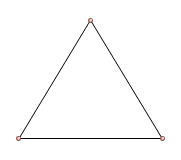```context.beginPath();
context.lineTo((canvasWidth + this.width)/2 + gamma, this.padding + this.height - gamma);
context.lineTo((canvasWidth - this.width)/2, this.padding + this.height + this.innerBorder);
context.closePath();```
```var trianglePath:GraphicsPath = new GraphicsPath(new Vector.(), new Vector.());
trianglePath.lineTo((canvasWidth + iconWidth)/2 + gamma, padding + iconHeight - gamma);
trianglePath.lineTo((canvasWidth - iconWidth)/2, padding + iconHeight + innerBorder);

Although beginning a path is slightly different, both JS and AS3 use the methods `moveTo` and `lineTo`.

To add a little complexity, let's round the corners of the triangle (and we are not going to take the stroke shortcut I used in in my previous article: HTML 5 Canvas Example).

To round the corners we will use Bézier curves, which are supported by both languages. The type of Bézier curve will be quadratic (opposed to cubic). Quadradtic Bézier curves have two anchor points; the curve of the line between them is defined by one control point (Cubic Bézier curves have two control points).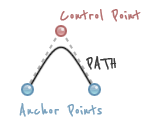Adding a pair of control points at each corner will give a rounded effect.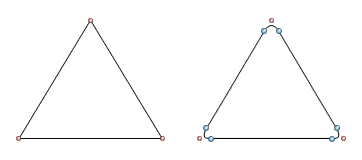```// Create the triangular path (with rounded corners)
context.beginPath();
// Top Corner
// Right Corner
context.lineTo((canvasWidth + this.width)/2 + gamma, this.padding + this.height - gamma);
context.quadraticCurveTo((canvasWidth + this.width)/2 + y, this.padding + this.height + this.innerBorder, (canvasWidth + this.width)/2, this.padding + this.height + this.innerBorder);
// Left Corner
context.lineTo((canvasWidth - this.width)/2, this.padding + this.height + this.innerBorder);
context.quadraticCurveTo((canvasWidth - this.width)/2 - y, this.padding + this.height + this.innerBorder, (canvasWidth - this.width)/2 - gamma, this.padding + this.height - gamma);
// Close Path
context.closePath();```
```// Create the triangular path (with rounded corners)
var trianglePath:GraphicsPath = new GraphicsPath(new Vector.(), new Vector.());
// Top Corner
// Right Corner
trianglePath.lineTo((canvasWidth + iconWidth)/2 + gamma, padding + iconHeight - gamma);
trianglePath.curveTo((canvasWidth + iconWidth)/2 + y, padding + iconHeight + innerBorder, (canvasWidth + iconWidth)/2, padding + iconHeight + innerBorder);
// Left Corner
trianglePath.lineTo((canvasWidth - iconWidth)/2, padding + iconHeight + innerBorder);
trianglePath.curveTo((canvasWidth - iconWidth)/2 - y, padding + iconHeight + innerBorder, (canvasWidth - iconWidth)/2 - gamma, padding + iconHeight - gamma);
// Close Path

JavaScript uses the method `quadraticCurveTo` on the HTML5 canvas to create the control point. ActionScript 3 uses the method `curveTo`.

## Create the Inner Stroke Path

Next we create a smaller triangle within our first triangle. Later we will stroke it and utilize standard properties to curve the border of our stroke for us.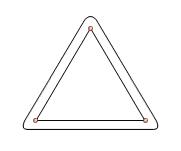```// Create the inner border path
context.beginPath();
context.lineTo((canvasWidth + this.width)/2 - this.lineWidth, this.padding + this.height - this.lineWidth/2);
context.lineTo((canvasWidth - this.width)/2 + this.lineWidth, this.padding + this.height - this.lineWidth/2);
context.closePath();```
```// Create the inner border path
var strokePath:GraphicsPath = new GraphicsPath(new Vector.(), new Vector.());
strokePath.lineTo((canvasWidth + iconWidth)/2 - innerBorder, padding + iconHeight - innerBorder/2);
strokePath.lineTo((canvasWidth - iconWidth)/2 + innerBorder, padding + iconHeight - innerBorder/2);

## Bang!

With any icon, we have a message. The warning standard is an exclamation point (aka a "bang" character) which we add in the center of our icon. We could use a text representation but we will not do that for a various reasons (the user might not have that font, the size cost for embedding an entire typeface just for one character is not worth it, etc.)```// Create the text (aka bang) path
context.beginPath();
// Top
context.moveTo(canvasWidth/2 - 8, this.padding + 45);
// Bottom
context.lineTo(canvasWidth/2 + 3, this.padding + 66);
// Close path
context.lineTo(canvasWidth/2 - 8, this.padding + 44);
```
```// Create the text (aka bang) path
var bangPath:GraphicsPath = new GraphicsPath(new Vector.(), new Vector.());
// Top
bangPath.moveTo(canvasWidth/2 - 8, padding + 45);
// Bottom
bangPath.lineTo(canvasWidth/2 + 3, padding + 66);
// Close path
bangPath.lineTo(canvasWidth/2 - 8, padding + 44);```

Again the major difference between the two approaches are the methods `quadraticCurveTo` versus `curveTo`.

## Exclamation 'Point'

To finish our bang character, we add a circle:```// Draw dot
var centerX = canvasWidth/2;
var centerY = this.padding + 84;
context.closePath();```
```// Draw Dot
var centerX:Number = canvasWidth/2;
var centerY:Number = padding + 84;

JavaScript and ActionScript have more efficient ways to create circles including the methods `arc` and `drawCircle` respectively.

## Draw Inside the Lines

The paths are complete: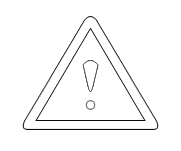In JavaScript and ActionScript and you won't actually see the path until assign a fill or stroke to them. The following code defines what the fills and strokes will look like:

```// Background Gradient Fill

// Text and Stroke Fill

// Stroke
context.lineWidth = this.lineWidth;
context.lineJoin = "round";
context.strokeStyle = bangFill;```
```// Background Gradient Fill
backFill.colors = [secondaryColor, tertiaryColor, primaryColor];
backFill.ratios = [iconHeight/2, iconHeight, iconHeight];
backFill.matrix = new Matrix();

// Text and Stroke Fill
bangFill.colors = [0x555555, 0x333333];
bangFill.matrix = new Matrix();

// Transparent Fill
var transparentFill:GraphicsSolidFill = new GraphicsSolidFill();
transparentFill.alpha = 0;

// Stroke
var stroke:GraphicsStroke = new GraphicsStroke(lineWidth);
stroke.joints = JointStyle.ROUND;
stroke.fill = bangFill;```

## Draw

```// Fill the background path
context.fillStyle = backFill;
context.fill();

// Stroke the inner border path
context.stroke();

// Fill the bang path
context.fillStyle = bangFill;
context.fill();	```
```// Fill and stroke all paths
var iconGraphics:Vector. = new Vector.();
iconGraphics.push(backFill, trianglePath, bangFill, bangPath, transparentFill, stroke, strokePath);
graphics.drawGraphicsData(iconGraphics);```

JavaScript applies the path fills on the HTML5 Canvas as each one is completed. ActionScript 3 can apply all path fills and strokes all at one time using the method `drawGraphicsData`.

We see the results below: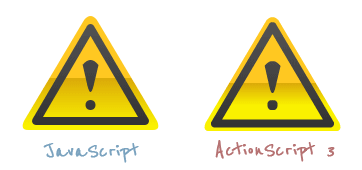We are almost there, but there is something missing. Let's add a subtle shadow.

```// Add a subtle shadow
```// Add a subtle shadow
filters = new Array(new DropShadowFilter(0, 0, 0x000000, 1, 10, 10));```

## Final Images